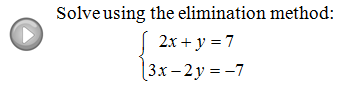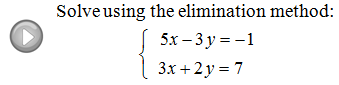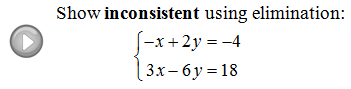## Pages

Showing posts with label linear. Show all posts
Showing posts with label linear. Show all posts

### Interactive: Slope-Intercept Form

Interactive Instructions: Slope-intercept form: y = mx + b. Move the green points to change m and b.

### Dynamic Quiz - Solving Basic Linear Equations

Test your skills and answer 5 questions correctly.

Try this! Your score: 0/5
Solve for the given variable.
$$2x+3=13$$
$$x=$$
Push the Check button for feedback.

### Chapter 4 Sample Test Questions

Click here for a worksheet containing 20 sample test questions with answers.

Example: Solve the system using the graphing method:

Example: Solve the system using the substitution method:

Example: Solve the system using the addition method:

Solve.
Interest Problem: Marsha has $4,500 saved up in two different accounts. She has a savings account earning 2% in annual interest and a CD earning 5% annual interest. How much does she have in each account if her total interest for the year was$198?

Answer: Marsha has $900 in savings and$3,600 in the CD.

Geometry Problem: The length of a rectangular garden is 3 feet less than twice the width. If the perimeter measures 54 feet then what are the dimensions of the garden?
Solve using the substitution method.
Distance Problem: Al can row his boat 30 miles downstream to the general store in 3 hours. The return trip, against the current will take him 5 hours. How fast is the river current?
Answer: The river current is flowing at 2 miles per hour.

Geometry Problem: Two angles are supplementary and one angle is 45 degrees less than 4 times the other. Find the two angles.
Graph all solutions to the system of inequalities:

### Solving Linear Systems by Elimination

When solving linear systems, the elimination method - sometimes called the addition method - usually is the method of choice.  This technique is completely algebraic and quick once you get the hang of it.  The idea is to eliminate one variable by adding equivalent equations together.

Solve the system using the elimination method:
Step 1: Multiply one or both of the equations by factors that will line up one variable to eliminate.
Step 2: Add the equations together.
Step 3: Back substitute and present the answer as an ordered pair.
Tip: If you multiply an equation by any number - remember to distribute!

Solve the system using the elimination method:
To eliminate the variable y, multiply the first equation by 3 and the second equation by 2.
Now add the equations together.

You will always have to back substitute to find the value of the other coordinate.
Solve the systems using the elimination method:
You will likely encounter systems that are not lined up in standard form.  In this case, you should first rearrange the equation before using the elimination method.
Clearing Fractions: If we are given an equation with fractional coefficients, we can clear them out by multiplying both sides by a common multiple of the denominator.  This is a handy technique which we will use often in our study of Algebra.  Do not abuse this, as it only works on equations and not expressions.
The LCM of the denominators is 30.
Distribute and then simplify.
No more fractions; now that is nice!
This gives equivalent equations.
Multiply both sides of any equation by the LCD to clear the fractional coefficients.

Solve the systems using the elimination method.
Video Examples on YouTube: